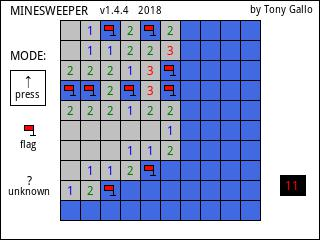Minehunt program
01-05-2014, 01:32 PM (This post was last modified: 01-06-2014 08:31 AM by LarsF.)
Post: #1
 LarsFJunior Member Posts: 39 Joined: Dec 2013
Minehunt program
From an idea from HP48G

Code:
 // Version 0.1 // Lars Fredriksson // 2013-2014 LOCAL rows,cols; DRAW(); MOVE(); CHECK_BOMBS(); CHK(); EXPORT Minehunt() BEGIN   LOCAL Key,Pos:=1,Tmp,Bombs,Moves:=0;     rows:=10;   cols:=14;   L0:=SORT(MAKELIST(RANDINT(rows*cols-3)+2,X,1,rows*cols*.2)); // make some bombs ca 20%   L1:=MAKELIST(0,X,1,rows*cols); // make board   L1(1):=1;      RECT_P();   Tmp:=Pos; //REMEMBER POS   Bombs:=CHECK_BOMBS(Pos);   DRAW(Pos,Bombs,0,"Bombs "+Bombs +" Moves "+Moves); // Draw board      REPEAT     FREEZE;     Key:=WAIT();           Pos:=MOVE(Key,Pos); // make a move     L1(Pos):=1;     //Have we moved     IF Tmp≠Pos THEN       Moves:=Moves+1;        Bombs:=CHECK_BOMBS(Pos);         // Check if we step on a bomb       IF POS(L0,Pos)≠0 THEN         DRAW(Pos,Bombs,1,"Sorry, you step on a bomb after "+Moves+" moves"); // draw board and reveal bombs         WAIT(−1);         BREAK;       END;       // check if we get to the goal       IF Pos==rows*cols THEN         DRAW(Pos,Bombs,1,"Congratulations, you made it in "+Moves+" moves");         WAIT(−1);         BREAK;        END;       DRAW(Pos,Bombs,0,"Bombs "+Bombs +" Moves "+Moves); // redraw board       Tmp:=Pos; //REMEMBER POS     END;   UNTIL Key==4; //ESC END; CHECK_BOMBS(P) BEGIN   LOCAL Count:=0;   LOCAL LE:=0,RE:=0; // EDGE   IF P MOD cols == 1 THEN     LE:=1;   END;   IF P MOD cols == 0 THEN     RE:=1;   END;   // count bombs in the neigbourhood   IF LE==0 THEN     Count:=Count+(POS(L0,CHK(P,P-cols-1))>0); //7      Count:=Count+(POS(L0,CHK(P,P-1))>0);      //4     Count:=Count+(POS(L0,CHK(P,P+cols-1))>0); //1   END;   Count:=Count+(POS(L0,CHK(P,P-cols))>0);   //8   Count:=Count+(POS(L0,CHK(P,P+cols))>0);   //2   IF RE==0 THEN      Count:=Count+(POS(L0,CHK(P,P-cols+1))>0); //9     Count:=Count+(POS(L0,CHK(P,P+1))>0);      //6     Count:=Count+(POS(L0,CHK(P,P+cols+1))>0); //3   END;       RETURN Count; END; CHK(T,A) BEGIN   IF A<1 THEN     RETURN T;   ELSE     RETURN A;   END; END; DRAW(P,B,S,T) BEGIN  LOCAL X,Y,X1,X2,Y1,Y2,C:=1;  // calculate size of boxes  X0:=IP(320/(cols+2));  Y0:=IP(240/(rows+2));  X1:=IP(320/(cols+2));  Y1:=IP(240/(rows+2));    RECT_P(0,0,320,Y1,RGB(255,255,255),RGB(255,255,255));  FOR Y:=1 TO rows DO    FOR X:=1 TO cols DO      IF L1(C)==1 THEN        IF C==P THEN          RECT_P(X*X0,Y*Y0,X*X0+X1,Y*Y0+Y1,RGB(0,0,0),RGB(128,255,128));        ELSE          RECT_P(X*X0,Y*Y0,X*X0+X1,Y*Y0+Y1,RGB(0,0,0),RGB(255,255,255));        END;      ELSE        RECT_P(X*X0,Y*Y0,X*X0+X1,Y*Y0+Y1,RGB(0,0,0),RGB(180,180,180));      END;      IF S == 1 THEN  //SHOW BOMBS        IF POS(L0,C)≠0 THEN          IF C==P THEN            RECT_P(X*X0,Y*Y0,X*X0+X1,Y*Y0+Y1,RGB(0,0,0),RGB(255,0,0));          ELSE            RECT_P(X*X0,Y*Y0,X*X0+X1,Y*Y0+Y1,RGB(0,0,0),RGB(255,180,180));          END;        END;      END;      C:=C+1;    END;  END;  TEXTOUT_P(T,10,5);   //BLIT_P(G1); END; MOVE(KEY,P) BEGIN     LOCAL LE:=0,RE:=0; // EDGE   IF P MOD cols == 1 THEN     LE:=1;   END;   IF P MOD cols == 0 THEN     RE:=1;   END;   CASE     IF KEY==32 THEN  //7       IF P-cols-1 >= 0 THEN          IF LE==0 THEN           P:=P-cols-1;         END;       END;      END;     IF KEY==33 THEN  //8       IF P-cols >= 0 THEN           P:=P-cols;        END;      END;     IF KEY==34 THEN  // 9       IF P-cols+1 >= 0 THEN         IF RE==0 THEN            P:=P-cols+1;         END;       END;      END;     IF KEY==37 THEN  //4       IF P-1 >= 0 THEN          IF LE==0 THEN           P:=P-1;         END;       END;      END;     IF KEY==39 THEN  //6       IF P+1 ≤ rows*cols THEN          IF RE==0 THEN           P:=P+1;         END;       END;      END;     IF KEY==42 THEN  //1       IF P+cols-1 < rows*cols THEN          IF LE==0 THEN           P:=P+cols-1;         END;       END;      END;     IF KEY==43 THEN  //2       IF P+cols ≤ rows*cols THEN          P:=P+cols;       END;      END;     IF KEY==44 THEN  //3       IF P+cols+1 ≤ rows*cols THEN          IF RE==0 THEN           P:=P+cols+1;         END;       END;      END;     DEFAULT   END;   RETURN P;  END;
01-05-2014, 04:07 PM
Post: #2Harold A ClimerJunior Member Posts: 13 Joined: Dec 2013
RE: Minehunt program
There is a syntax error in Line 108.
Can not figure out what the problem is,yet
01-05-2014, 04:13 PM (This post was last modified: 01-05-2014 04:14 PM by LarsF.)
Post: #3
 LarsFJunior Member Posts: 39 Joined: Dec 2013
RE: Minehunt program
Should be L( C )
Post: #4Terje VallestadMember Posts: 152 Joined: Dec 2013
RE: Minehunt program
(01-05-2014 04:13 PM)LarsF Wrote:  Should be L( C )

You are sure it should not be L1(C) rather than L(C)? I get a Syntax error with L(C) whilst L1(C) compiles fine....

Edit: I noted the forum software changes "L1( C )" to L1(C) Copyright which is unintended. Rewritten with a space either side of the C that should not be in the program.

Cheers, Terje
01-05-2014, 10:22 PM (This post was last modified: 01-05-2014 10:25 PM by LarsF.)
Post: #5
 LarsFJunior Member Posts: 39 Joined: Dec 2013
RE: Minehunt program
L1( C ) should it be, thanks. I should have use the code tag, note the quote tag, I believe i would look right then with spaces and no copyright signs...
01-05-2014, 11:24 PM
Post: #6
 tgalloJunior Member Posts: 47 Joined: Dec 2013
RE: Minehunt program
If you are interested I wrote a version.

HP PRIME PROGRAMS01-06-2014, 08:35 AM
Post: #7
 LarsFJunior Member Posts: 39 Joined: Dec 2013
RE: Minehunt program
I manage to change it to a code tag, looks much better now :-)